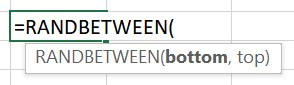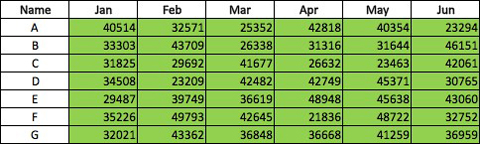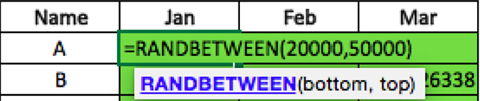RANDBETWEEN Formula in Excel
Friday, Jul 10# How to Use the RANDBETWEEN Formula in Excel

The RANDBETWEEN formula is used to insert random numeric values according to your specification between a bottom and top threshold.

Note that every time you refresh the screen, the data will randomize. Therefore, if you are looking to randomize data once and then store it then the best practice is to randomize the data, copy it, and paste special the values somewhere else in the worksheet.

Formula explanation:

• Bottom: The minimum value of our requirement.
• Top: The maximum value of our requirement.Example:

In this example, we are going to insert random values between 20000 to 50000 into a data table below.

=RANDBETWEEN(20000,50000)

Solution:*Note the tables above are only a sample of an example with few data entries, and the formulas are based on the whole population of raw data which are not reflected in the example.

Formula Implementation:You can set the parameters to the numerical values as a bottom and top threshold as you see fit as you prefer but this is just one example of a case between 20,000 and 50,000.

I hope that helps. Please leave a comment below with any questions or suggestions. For more in-depth Excel training, checkout our Ultimate Excel Training Course here. Thank you!

Article RatingInline Feedbacks